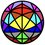# Temperature for small systems

From the Maxwell-Boltzmann distribution we know that :

$v_{\text{rms}}^{2}=\frac{3RT}{M}$

Labelling all particles of the gas with an index we know that :

$v_{\text{rms}}^{2}=\dfrac{\displaystyle\sum_{i=1}^{n} v_i^{2}}{n}$

where n is the number of particles in the gas

So making $T$ the subject of the equation we get :

$T= \dfracM \sum_{i=1}^{n} v_i^{2}}{3Rn}$

This is assuming the case that the system containing our gas is not in motion, if in motion we should be taking the velocities deviating from the velocity of the centre of mass of the gas

Keeping the average velocity as $\overline v$

Our new equation to combat moving systems should be

$T= \dfracM \sum_{i=1}^{n} (v_i-\overline v)^{2}}{3Rn}$

$\text{Both } v_i \text{ and } \overline v \text{ are to be treated as vectors}$

This can be applied to smaller systems easily, so I was wondering whether this is a good argument or not, as I have heard you can’t define a temperature for smaller systems

If true, will such systems obey the law of thermodynamics, as in my sight there are just statistical laws which are more true as the size of the system increasesNote by Jason Gomez
4 months, 4 weeks ago

This discussion board is a place to discuss our Daily Challenges and the math and science related to those challenges. Explanations are more than just a solution — they should explain the steps and thinking strategies that you used to obtain the solution. Comments should further the discussion of math and science.

When posting on Brilliant:

• Use the emojis to react to an explanation, whether you're congratulating a job well done , or just really confused .
• Ask specific questions about the challenge or the steps in somebody's explanation. Well-posed questions can add a lot to the discussion, but posting "I don't understand!" doesn't help anyone.
• Try to contribute something new to the discussion, whether it is an extension, generalization or other idea related to the challenge.

MarkdownAppears as
*italics* or _italics_ italics
**bold** or __bold__ bold
- bulleted- list
• bulleted
• list
1. numbered2. list
1. numbered
2. list
Note: you must add a full line of space before and after lists for them to show up correctly
paragraph 1paragraph 2

paragraph 1

paragraph 2

[example link](https://brilliant.org)example link
> This is a quote
This is a quote
    # I indented these lines
# 4 spaces, and now they show
# up as a code block.

print "hello world"
# I indented these lines
# 4 spaces, and now they show
# up as a code block.

print "hello world"
MathAppears as
Remember to wrap math in $$ ... $$ or $ ... $ to ensure proper formatting.
2 \times 3 $2 \times 3$
2^{34} $2^{34}$
a_{i-1} $a_{i-1}$
\frac{2}{3} $\frac{2}{3}$
\sqrt{2} $\sqrt{2}$
\sum_{i=1}^3 $\sum_{i=1}^3$
\sin \theta $\sin \theta$
\boxed{123} $\boxed{123}$

Sort by:

@Siddharth Chakravarty Have any idea about this? Whether it could be right/wrong, or know someone who will know ?

- 4 months, 3 weeks ago

Im still yet to complete Thermodynamics completely, I cant mention the people I know ugh.

- 4 months, 3 weeks ago

Why?

- 4 months, 3 weeks ago

Is it because you know no one who would be interested in this, or is it because the people you know(who would know about this) won’t be interested in this

- 4 months, 3 weeks ago

No, no actually when we try to mention a person we can see the name of the person pop-up, and thus clicking on it, the person gets mentioned but when I am trying to mention those people I can't see their name pop-up.

- 4 months, 3 weeks ago

Yeah you need to find them somewhere they commented and use Frisk’s technique, that is why I made a new note for keeping mention ids

- 4 months, 3 weeks ago

now that is gonna be a long process, I will give you the names rather Josh Silverman, Aryan Sanghi, Alakh Bhattacharya, Agnishom Chattopadhaya, etc.

- 4 months, 3 weeks ago

Ok I think I know where to find Aryan Sanghi and Alakh Bhattacharya

- 4 months, 3 weeks ago

Couldn’t find the others

- 4 months, 3 weeks ago

Who do you want to mention btw?

- 4 months, 3 weeks ago

- 4 months, 3 weeks ago

The laws of thermodynamics are universal, they could be applied to every system, big or small.

Considering the last equation you wrote for $T$, it is based on Kinetic Theory of Gases which is applicable only for large systems, so the last equation might differ a good amount from experimental value if applied to small systems. Also, temperature is defined for all systems, big or small, but the only problem is that Kinetic theory of gases is not valid for small systems, so you can't define temperature for small systems using KTG. Hope it helps. :)

- 4 months, 3 weeks ago

Thank you, this helps a lot

- 4 months, 3 weeks ago